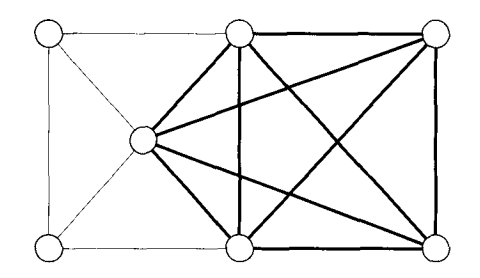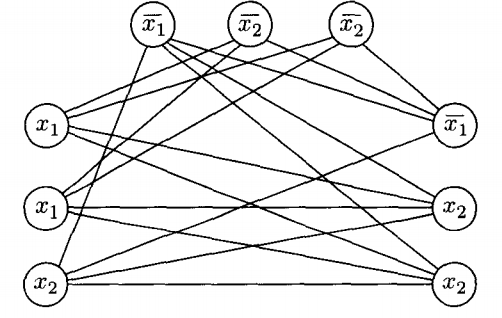# NP-Completeness

## Overview

Recall that $P$ is the set of problems which can be solved efficiently, and that $NP$ is the set of problems which can be verified efficiently.

Also recall that it is unknown whether $P$ is a subset of $NP$, or whether the two classes are equal:There is another class of problems, called $NP$-complete. These are the hardest problems of $NP$, without any known polynomial time solution. If any polynomial time algorithm for an $NP$-complete problem were found, it would imply that $P = NP$.

The concept of $NP$-completeness is important for two reasons:

1. It is important in the question of $P$ and $NP$. $NP$-complete problems represent those we suspect to be in $NP$ but not in $P$.
2. It can prevent you from spending time searching for an efficient algorithm to some problem. If the problem can be shown to be $NP$-complete, it is very unlikely an efficient algorithm exists.

## Polynomial Reducibility

$NP$-completeness relies on a concept called polynomial reducibility.

Recall that if some problem $A$ reduces to some problem $B$, then a solution to $B$ can be used to solve $A$. We used reducibility to help prove the undecidability of certain problems.

Polynomial reducibility is a reduction that takes efficiency into account. If $A$ polynomially reduces to $B$, then $B$ can be used to solve $A$ with only a polynomial increase in time.

Formally, $f: \Sigma^\ast \rightarrow \Sigma^\ast$ is a polynomial time function if some polynomial time Turing machine $M$ exists that halts with $f(w)$ on its tape when started on input $w$.

$A$ is polynomial time reducible to $B$, written $A \le_P B$ if some polynomial time function $f$ exists, where for every $w$,

$w \in A \Longleftrightarrow f(w) \in B$.It is identical to general reducibility, with the exception that the function $f$ must compute in polynomial time.

## NP-Completeness

A language $B$ is $NP$-complete if it satisfies the following:

1. $B$ is in $NP$.
2. Every language $A$ in $NP$ is polynomial time reducible to $B$.

$NP$-complete problems are the hardest ones in $NP$. If a given $NP$-complete problem had an efficient polynomial solution, by definition, we would also have an efficient polynomial solution to every problem in $NP$.

Hence, a polynomial time solution to an $NP$-complete problem would imply $P = NP$. Of course no efficient solution to any $NP$-complete problem is known.

To show that a given problem $B$ is $NP$-complete, we must first show that it is in $NP$, and also show one of the following two things:

1. Every problem in $NP$ is polynomial time reducible to $B$.
2. Show that some other $NP$-complete problem $C$ polynomially reduces to $B$.

The second is sufficient since, if we know that $C$ is $NP$-complete, then all problems in $NP$ can be polynomially reduced to $C$. If we can show that $C$ polynomially reduces to $B$, then we also shown all problems in $NP$ polynomially reduce to $B$ since doing the two polynomial reductions in sequence forms another polynomial reduction.

In order to use approach 2, we need to have some first $NP$-complete problem which is shown to be $NP$-complete via approach 1. That problem is the $SAT$ problem.

## The SAT Problem

The $SAT$ problem deals with the satisfiability of boolean expressions. These boolean expressions are composed of variables, the binary operators and ($\wedge$), or ($\vee$), not (represented with bars over variables).

For example the following is a boolean expression:

$(\overline{x} \wedge y) \vee (x \wedge \overline{z})$

What variable assignments would result in the expression being satisfied - being true?

Some boolean expressions are not satisfiable. For example:

$(\overline{x} \wedge y) \wedge (x \wedge \overline{z})$

The $SAT$ problem can be given as:

$SAT = \{E\; |\; E \text{ is a boolean expression that is satisfiable}\}$.

Given some boolean expression, we would need to find a variable assignment resulting in the expression being satisfied.

The $SAT$ problem is in $NP$. How could we show this?

## The Cook-Levin Theorem

The Cook-Levin theorem is as follows:

All problems in $NP$ are polynomially reducible to $SAT$.

This, along with $SAT \in NP$, tells us that $SAT$ is an $NP$-complete problem.

The proof of the Cook-Levin theorem is quite complex, but the basic idea is as follows:

1. Given some problem $A$ which is in $NP$, we know there is a non-deterministic Turing machine $N$ which decides $A$ in polynomial time.
2. We take the input to $N$, $w$, and build a boolean expression which simulates $N$ on $w$.
3. If the machine $N$ accepts $w$, then the expression has a satisfying assignment of variables (which will correspond to the branch of computation that accepts).
4. If the machine $N$ rejects $w$, then the expression has no satisfying assignment.
5. We could then use a solution to $SAT$ in order to decide whether or not $N$ accepts $w$ - which will decide the problem $A$.

Using this technique, we can build an instance of the $SAT$ problem which corresponds to any problem in $NP$. Therefore all problems in $NP$ are reducible to $SAT$. The reduction is also a polynomial reduction because the technique takes only a polynomial amount of time.

The physical computers that we use are made up of only logical operations. All programs that we run are efficiently mapped onto this hardware. The fact that we can design a logical expression to simulate a Turing machine should not be too surprising.

The Cook-Levin theorem is important because it provides us with the first $NP$-complete problem. Other problems can be shown to be $NP$-complete by reducing $SAT$ to them.

## The 3SAT Problem

$3SAT$ is a special case of the $SAT$ problem where the expressions must be in a special form.

A literal is either a single variable or a negated single variable. A clause is several literals connected with ors. An expression is in conjunctive normal form (cnf) if it is composed of multiple clauses connected with ands.

The expressions built by the Cook-Levin theorem are in cnf.

An expression is in 3-cnf if each clause has exactly three literals.

The $3SAT$ problem is given as:

$3SAT = \{E\; |\; E \text{ is a satisfiable 3-cnf expression}\}$.

$3SAT$ is also an $NP$-complete problem. This follows from the implementation detail of the Cook-Levin theorem which produces boolean expressions that are in cnf.

These expressions can be converted from arbitrary cnf to 3-cnf as follows:

1. If a clause has fewer than 3 literals, expand it to 3 by repeating a literal. For example $(x \vee y)$ can replaced with $(x \vee y \vee y)$ which does not change the meaning.
2. If a clause has more than 3 literals, replace it with several clauses adding variables such that the meaning is preserved. For example, $(a_1 \vee a_2 \vee a_3 \vee a_4)$ can be replaced with $(a_1 \vee a_2 \vee z) \wedge (\overline{z} \vee a_3 \vee a_4)$. The new variable $z$ works as a "selector" - if one of $a_1$ or $a_2$ is true, $z$ will be false. If $a_3$ or $a_4$ is true, $z$ will be true. If multiple are true, it does not matter what $z$ is.

Thus the restriction of being in 3-cnf does not change the fact that satisfiability is $NP$-complete.

$3SAT$ is useful because it can be used in reductions for other problems.

## The Clique Problem

Recall that a clique is a fully-connected sub-graph within a graph. A $k$-clique is a clique with $k$ nodes.

The following graph has a 5-clique:We can state the clique problem as:

$CLIQUE = \{\langle G, k \rangle\; |\; G \text{ is an undirected graph with a } k\text{-clique}\}$.

We will now show that the $CLIQUE$ problem is $NP$-complete. To do so, we first need to show that $CLIQUE$ is in $NP$. How could we do this?

We would next need to show that all problems in $NP$ are polynomial reducible to $CLIQUE$, as we did with $SAT$ or $3SAT$.

Instead of showing this directly, we can show that $3SAT$ is polynomial reducible to $CLIQUE$. Because $3SAT$ is in $NP$-complete, if it reduces to $CLIQUE$, $CLIQUE$ would also be $NP$-complete.

How can we reduce $3SAT$ to $CLIQUE$? Graphs and boolean expressions seem unrelated, but there is a way to do this.

We convert a boolean expression with $k$ clauses such as:

$E = (a_1 \vee b_1 \vee c_1) \wedge (a_2 \vee b_2 \vee c_2) ... \wedge (a_k \vee b_k \vee c_k)$

Into an instance of the $k-CLIQUE$ problem. For the reduction, we organize the graph into $k$ groups of three nodes called triplets. Each triplet corresponds to one clause of $E$. We label each node with the literal it corresponds to in $E$.

We connect all nodes in the graph except for the following cases:

1. No edge is present between nodes in the same triple.
2. No edge is present between nodes with contradictory labels, e.g. $x$ and $\overline{x}$.

For example, the expression $(x_1 \vee x_1 \vee x_2) \wedge (\overline{x_1} \vee \overline{x_2} \vee \overline{x_2}) \wedge (\overline{x_1} \vee x_2 \vee x_2)$ would be converted into the following graph:This works because, for the expression to be true, at least one literal in each clause must be true. For the graph to have a $k$ clique, it must have a node in each triple as part of the clique.

There can be no logical contradictions in the clique because no contradictory nodes are connected.

This reduction also would take polynomial time. Therefore $3SAT \le_P CLIQUE$ which implies $CLIQUE$ is $NP$-complete.